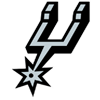2021 NBA MVP Odds
+475
4.75 to 1Luka Doncic
12.8% implied probability

+600
6 to 1Stephen Curry
10.5% implied probability

+650
6.5 to 1Giannis Antetokounmpo
9.8% implied probability

+650
6.5 to 1Kevin Durant
9.8% implied probability

+1000
10 to 1Joel Embiid
6.7% implied probability

+1500
15 to 1Nikola Jokic
4.6% implied probability

No changes have been recorded yet.
+1800
18 to 1LeBron James
3.9% implied probability

+2000
20 to 1Damian Lillard
3.5% implied probability

+2000
20 to 1James Harden
3.5% implied probability

No changes have been recorded yet.
+2500
25 to 1Trae Young
2.8% implied probability

+3000
30 to 1Jayson Tatum
2.4% implied probability

No changes have been recorded yet.
+3500
35 to 1Devin Booker
2.0% implied probability

+3500
35 to 1Anthony Davis
2.0% implied probability

+3500
35 to 1Ja Morant
2.0% implied probability

+3500
35 to 1Donovan Mitchell
2.0% implied probability

+3500
35 to 1Paul George
2.0% implied probability

+5000
50 to 1Julius Randle
1.4% implied probability

+5500
55 to 1Zach LaVine
1.3% implied probability

+7000
70 to 1LaMelo Ball
1.0% implied probability

+8000
80 to 1Domantas Sabonis
0.9% implied probability

+8000
80 to 1Chris Paul
0.9% implied probability

No changes have been recorded yet.
+8000
80 to 1Jaylen Brown
0.9% implied probability

+8000
80 to 1Karl-Anthony Towns
0.9% implied probability

+8000
80 to 1Bradley Beal
0.9% implied probability

+8000
80 to 1Jimmy Butler
0.9% implied probability

+9000
90 to 1Zion Williamson
0.8% implied probability

+10000
100 to 1Russell Westbrook
0.7% implied probability

+15000
150 to 1Kyrie Irving
0.5% implied probability

+15000
150 to 1Anthony Edwards
0.5% implied probability

No changes have been recorded yet.
+15000
150 to 1Bam Adebayo
0.5% implied probability

+18000
180 to 1De'Aaron Fox
0.4% implied probability

No changes have been recorded yet.
+20000
200 to 1Khris Middleton
0.4% implied probability

+20000
200 to 1Ben Simmons
0.4% implied probability

No changes have been recorded yet.
+25000
250 to 1Christian Wood
0.3% implied probability

No changes have been recorded yet.
+25000
250 to 1Michael Porter
0.3% implied probability

No changes have been recorded yet.
+25000
250 to 1CJ McCollum
0.3% implied probability

+30000
300 to 1DeMar DeRozan
0.2% implied probability

+30000
300 to 1Kawhi Leonard
0.2% implied probability

+30000
300 to 1Deandre Ayton
0.2% implied probability

+30000
300 to 1Tobias Harris
0.2% implied probability

+30000
300 to 1Nikola Vucevic
0.2% implied probability

+30000
300 to 1Brandon Ingram
0.2% implied probability

+30000
300 to 1Lonzo Ball
0.2% implied probability

+30000
300 to 1Malcolm Brogdon
0.2% implied probability

+30000
300 to 1Pascal Siakam
0.2% implied probability

+35000
350 to 1Collin Sexton
0.2% implied probability

No changes have been recorded yet.
+35000
350 to 1Cade Cunningham
0.2% implied probability

No changes have been recorded yet.
+40000
400 to 1Rudy Gobert
0.2% implied probability

No changes have been recorded yet.
+40000
400 to 1Kemba Walker
0.2% implied probability

No changes have been recorded yet.
+40000
400 to 1John Collins
0.2% implied probability

No changes have been recorded yet.
+40000
400 to 1Clint Capela
0.2% implied probability

No changes have been recorded yet.
+50000
500 to 1Tyrese Haliburton
0.1% implied probability

No changes have been recorded yet.
+50000
500 to 1Jrue Holiday
0.1% implied probability

+50000
500 to 1Kyle Lowry
0.1% implied probability

+50000
500 to 1Norman Powell
0.1% implied probability

No changes have been recorded yet.
+50000
500 to 1Draymond Green
0.1% implied probability

No changes have been recorded yet.
+50000
500 to 1Shai Gilgeous-Alexander
0.1% implied probability

+50000
500 to 1D'Angelo Russell
0.1% implied probability

No changes have been recorded yet.
+50000
500 to 1Victor Oladipo
0.1% implied probability

No changes have been recorded yet.
+50000
500 to 1Fred VanVleet
0.1% implied probability

+50000
500 to 1Aaron Gordon
0.1% implied probability

No changes have been recorded yet.
+50000
500 to 1Dejounte Murray
0.1% implied probability

No changes have been recorded yet.
+50000
500 to 1Jerami Grant
0.1% implied probability

No changes have been recorded yet.
+50000
500 to 1Kristaps Porzingis
0.1% implied probability